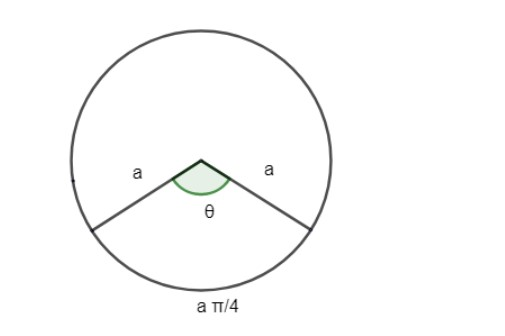Courses
Courses for Kids
Free study material
Free LIVE classes
MoreLIVE
Join Vedantu’s FREE Mastercalss

# Find the angle subtended at the center of a circle of radius ‘a’ by an arc of length $\left( \dfrac{a\pi }{4} \right)$cm.Verified
360.3k+ views
Hint:.The arc is any portion of the circumference of a circle. Arc length is the distance from one endpoint of the arc to the other point. Use an equation to find arc length $=2\pi r\left( \dfrac{\theta }{360} \right)$.

Given that the radius of the circle = a
We need to find the angle subtended by arc length $\left( \dfrac{a\pi }{4} \right)$From the figure it is clear that the length of arc is$\Rightarrow \dfrac{a\pi }{4}$
We need to find $\theta$.
The formula for finding the arc length is given by
$\Rightarrow$arc length$=2\pi r\left( \dfrac{\theta }{360} \right)$
We can find the arc length or portion of the arc in the circumference, if we know at what portion of 360 degrees the arc’s central angle is.
Arc length$=2\pi r\left( \dfrac{\theta }{360} \right)$, where r is the radius of the circle.
$\therefore 2\pi r\left( \dfrac{\theta }{360} \right)=\dfrac{a\pi }{4}$
We have been given arc length$=\dfrac{a\pi }{4}$
$\Rightarrow 2\pi a\left( \dfrac{\theta }{360} \right)=\dfrac{a\pi }{4}$
\begin{align} & 2\pi a\left( \dfrac{\theta }{360} \right)=\dfrac{a}{4}\pi \\ & \Rightarrow \dfrac{\theta }{360}=\dfrac{1}{8}\Rightarrow \theta =\dfrac{360}{8}={{45}^{\circ }} \\ \end{align}
$\therefore$We get the angle subtended at the center of the circle$={{45}^{\circ }}$
Note: Here, arc length $=\dfrac{a\pi }{4}$
If we assume value of $\theta ={{45}^{\circ }}$and applying we get
\begin{align} & =2\pi a\left( \dfrac{45}{360} \right) \\ & =2\pi a\left( \dfrac{1}{8} \right)=\dfrac{a\pi }{4} \\ \end{align}